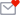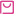收藏

# 必须要掌握的基础中医方剂之间的关系与演变

1. 麻黄汤

531. （麻黄、桂枝、杏仁、甘草）

1. 麻黄加术汤=麻黄汤+白术

2. 麻杏苡甘汤=麻黄汤-桂枝+薏苡仁

3. 麻杏石甘汤=麻黄汤-桂枝+石膏

（1） 越婢汤=麻杏石甘汤-杏仁+生姜、大枣

（2） 大青龙汤=麻黄汤+麻杏石甘汤+生姜、大枣

4. 三拗汤=麻黄汤-桂枝

（1） 华盖散=三拗汤+陈皮、桑皮、苏子、茯苓

（2） 冷哮丸=三拗汤+南星、细辛、白矾、猪牙皂、生姜、半夏、乌头、款冬花、紫苑、川椒

（3） 定喘汤=三拗汤+桑皮、半夏、款冬花、苏子、黄苓、白果

（1） 二、桂枝汤

532. （桂枝、芍药、甘草、生姜、大枣）

1. 桂枝加葛根汤=桂枝汤+葛根

2. 葛根汤=桂枝汤+葛根、麻黄

3. 黄芪桂校五物汤=桂枝汤-甘草+黄芪

4. 小青龙汤=桂枝汤-生姜、大枣+干姜、细辛、麻黄、半夏、五味子

5. 再造散=桂枝汤+人参、黄芪、附子、川芎、细辛、防风、羌活

6. 桂枝芍药知母汤=桂枝汤-大枣+知母、防风、白术、附子

7. 当归四逆汤=桂枝汤-生姜+当归、细辛、通草

533. 当归四逆加生姜吴茱萸汤=当归四逆汤+生姜、吴茱萸

8. 小建中汤=桂枝汤+饴糖

（1） 黄芪建中汤=小建中汤+黄芪

（2） 当归建中汤=小建中汤+当归

（1） 三、葱豉汤

534. （葱白、香豉）

1. 辛温解表法=葱豉汤+防风、桔梗、杏仁、陈皮

2. 葱豉桔梗汤=葱豉汤+竹叶、连翘、栀子、薄荷、甘草」

535. 3加减葳蕤汤=葱豉汤+葳蕤、白薇、甘草、葱白、香豉、薄荷、桔梗、大枣

（1） 四、香苏散

536. （香附、紫苏、陈皮、甘草）

1. 香苏葱豉汤=香苏散+葱豉汤

2. 加味香苏散=香苏散+荆芥、防风、秦艽、蔓荆子、川芎、生姜

3. 天香正气散=香苏散-甘草+干姜、乌药

（1） 五、败毒散

537. （前胡、羌活、独活、柴胡、枳壳、人参、桔梗、川芎、生姜、薄荷、甘草、茯苓《小儿药证直诀》）

1. 活人败毒散=败毒散-薄荷

2. 仓廪汤=败毒散+陈仓米

3. 荆防败毒散=败毒散-人参、生姜、薄荷+荆芥、防风。

（1） 银翘败毒散=荆防败毒散-荆芥、防风+金银花、连翘。

（2） 硝黄败毒散=荆防败毒散-荆芥、防风+芒硝、大黄

1. 六、大承气汤

538. （大黄、厚朴、枳实、芒硝）

539. 1黄龙汤=大承气汤+人参、当归、甘草

3. 三一承气汤=大承气汤-枳实+当归、甘草

540. 3小承气汤=大承气汤-芒硝

（1） 厚朴三物汤=小承气汤重用厚朴

（2） 厚朴七物汤=小承气汤+桂枝汤-芍药

（3） 麻仁丸=小承气汤+麻仁、杏仁、芍药、白蜜

（4） 承气养营汤=小承气汤+四物汤-川芎+知母

541. 4调胃承气汤=大承气汤-枳实、厚朴+甘草

（1） 凉膈散=调胃承气汤+竹叶、连翘、栀子、薄荷、黄芩

（2） 增液承气汤=调胃承气汤+增液汤-甘草

（3） 新加黄龙汤=调胃承气汤+人参、当归、姜汁、海参

（4） 玉烛散=调胃承气汤+四物汤

（5） 导赤承气汤=调胃承气汤-甘草+黄连、黄柏、生地黄、赤芍

2. 七、小柴胡汤

542. （柴胡、黄苓、人参、半夏、甘草、生姜、大枣）

1. 柴胡陷胸汤=小柴胡汤-人参、甘草、大枣+小陷胸汤（黄连、半夏、瓜蒌）

2. 大柴胡汤=小柴胡汤-人参、甘草+大黄、枳实、芍药

3. 柴胡枳桔汤：小柴胡汤-人参、甘草、生姜、大枣+枳壳、桔梗

4. 清脾饮：小柴胡汤-人参、大枣+草果仁、厚朴、青皮、白术、茯苓

5. 柴平汤=小柴胡汤+平胃散（苍术、厚朴、陈皮、甘草）

1. 八、芍药甘草汤

543. （芍药、甘草）

1. 四逆散=芍药甘草汤+柴胡、枳实

（1） 柴胡疏肝散=四逆散+川芎、香附、陈皮（一方无陈皮）

（2） 柴胡疏肝饮=柴胡疏肝散+青皮

544. 2.逍遥散＝芍药甘草汤＋柴胡、当归、白术、茯苓、生姜、薄荷

（1） 黑逍遥散：逍遥散+熟地黄

545. （2）丹栀逍遥散=逍遥散+牡丹皮、栀子

1. 九、半夏泻心汤

546. （半夏、甘草、人参、生姜、黄芩、黄连、大枣）

1. 生姜泻心汤=半夏泻心汤干姜减量+生姜

2. 甘草泻心汤=半夏泻心汤重用甘草（一方无人参）

3. 黄连汤=半夏泻心汤-黄芩+桂枝

1. 十、白虎汤

547. （知母、石膏、粳米、甘草）

1. 白虎加人参汤=白虎汤+人参

2. 白虎加桂枝汤=白虎汤+桂枝

3. 白虎加苍术汤=白虎汤+苍术

4. 化斑汤=白虎汤+玄参、犀角

5. 竹叶石膏汤=白虎汤-知母+竹叶、人参、半夏、麦冬

1. 十一、香薷散（三物香薷饮）

548. （香薷、厚朴、扁豆）

1. 新加香薷饮=香薷散+金银花、连翘

2. 黄连香薷饮：香薷散-扁豆（一方有扁豆、甘草）+黄连

3. 四味香薷饮=香薷散+黄连

4. 五物香薷饮=香薷散+获苓、甘草

549. 5.六味香薷饮＝五物香薷饮＋木瓜

550. 6.十味香薷饮＝六味香薷饮＋人参、黄芪、白术、陈皮

1. 十二、六一散

551. （滑石、甘草）

1. 益元散＝六一散＋辰砂

2. 碧玉散＝六一散＋青黛

3. 鸡苏散＝六一散＋薄荷

4. 玉泉散＝六一散＋寒水石

2. 十三、理中汤

552. （人参、白术、干姜、甘草）

1. 四君子汤＝理中汤－干姜＋茯苓

2. 枳实消痞丸＝理中汤＋四君子汤、枳实、厚朴、半夏、麦芽

3. 桂枝人参汤＝理中汤＋桂枝

4. 砂半理中汤＝理中汤＋砂仁、半夏

5. 丁萸理中汤＝理中汤＋丁香、吴茱萸6. 附子理中汤＝理中汤＋附子

7. 桂附理中汤＝理中汤＋附子、桂枝

8. 连理汤＝理中汤＋黄连

9. 理苓汤＝理中汤＋五苓散

2. 十四、四逆汤

553. （人参、白术、干姜、甘草）

1. 通脉四逆汤＝四逆汤重用附子、干姜

2. 白通汤=四逆汤-甘草+葱白

3. 四逆加人参汤=四逆汤+人参

（1） 四味回阳饮=四逆加人参汤

（2） 六味回阳饮=四味回阳饮+熟地黄、白术

（3） 千金温脾汤=四逆加人参汤+大黄（一方为四逆加人参

554. 汤+当归、芒硝；一方为四逆加人参汤-甘草+桂心）

5. 回阳救急汤=四逆汤+六君子汤、肉桂、麝香、五味子

6. 浆水散=四逆汤+肉桂、半夏、高良姜

555. 6.《本事方》温脾汤=四逆汤+厚朴、大黄、桂心

7. 实脾饮=四逆汤+白术、生姜、木香、槟榔、厚朴、草果仁、木瓜、大枣、茯苓

1. 十五、四君子汤

556. （人参、白术、茯苓、甘草）

1. 六神散=四君子汤+黄芪、扁豆（或黄芪、枳实）

2. 异功散=四君子汤+陈皮

3. 参苓白术散=异功散+薏苡仁、山药、莲米、扁豆、砂仁、桔梗

4. 归脾汤=四君子汤+黄芪、当归、桂圆肉、远志、酸枣仁、木香

5. 六君子汤=四君子汤+二陈汤（陈皮、半夏、茯苓、甘草）

（1） 柴芍六君子汤=六君子汤+柴胡、白芍

（2） 香砂六君子汤=六君子汤+木香、砂仁

（3） 健脾丸=香砂六君子汤-半夏+山楂、神曲、麦芽、山药、肉豆蔻、黄连

6. 八珍汤=四君子汤+四物汤

2. 十六、四物汤

557. （熟地黄、白芍、当归、川芎）

1. 圣愈汤=四物汤+黄芪、人参

2. 胶艾汤=四物汤+阿胶、艾叶

3. 地骨皮饮=四物汤+地骨皮、牡丹皮

4. 芩连四物汤=四物汤+黄芩、黄连

5. 玉烛散=四物汤+调胃承气汤

6. 桃红四物汤=四物汤+桃仁、红花

（1） 补阳还五汤=桃红四物汤-熟地黄+地龙、黄芪

（2） 血府逐瘀汤=桃红四物汤+四逆散、桔梗、牛膝

（3） 膈下逐瘀汤=挑红四物汤-熟地黄+延胡索、五灵脂、牡丹皮、枳壳、香附、甘草、乌药

6. 八珍汤=四物汤+四君子汤

2. 十七、八珍汤

558. （四君子汤+四物汤）

1. 八珍益母丸=八珍汤+益母草

2. 薯蓣丸=八珍汤+桂枝汤-生姜+干姜、豆卷、神曲、阿胶、麦冬、柴胡、防风、白薇、杏仁、桔梗

3. 独活寄生汤=八珍汤-白术+独活、桑寄生、秦艽、防风、细辛、杜仲、牛膝、桂心

559. 三痹汤=独活寄生汤-桑寄生+黄芪、续断

4. 十全大补汤=八珍汤+黄芪、肉桂

（1） 人参养营丸=十全大补汤-川芎+陈皮、五味子、远志

（2） 泰山磐石散=十全大补汤-肉桂+续断、砂仁、糯米、黄芩

2. 十八、炙甘草汤

560. （又名复脉汤：炙甘草、麻仁、大枣、生姜、桂枝、生地黄、人参、阿胶、麦冬、白酒）

1. 加减复脉汤=炙甘草汤-人参、大枣、生姜、桂枝、白酒+白芍

2. 大定风珠=加减复脉汤+牡蛎、鳖甲、龟板、鸡子黄、五味子

3. 一甲复脉汤=加减复脉汤——麻仁+牡蛎

4. 二甲复脉汤=一甲复脉汤+鳖甲

5. 三甲复脉汤=二甲复脉汤+龟板

2. 十九、六味地黄丸

561. （熟地黄、山药、山茱萸、茯苓、泽泻、牡丹皮）

1. 知柏地黄丸=六味地黄丸+知母、黄柏

2. 杞菊地黄丸=六味地黄丸+枸杞、菊花

3. 归芍地黄丸=六味地黄丸+当归、白芍

4. 参麦地黄丸==六味地黄丸+人参、麦冬

5. 七味都气丸=六味地黄丸+五味子

6. 八仙长寿丸（麦味地黄丸）=都气丸+麦冬

7. 左归丸=六昧地黄丸-茯苓、泽泻、牡丹皮+菟丝子、枸杞、牛膝、鹿胶、龟胶左归饮=六味地黄丸-泽泻、牡丹皮+枸杞、甘草

8. 肾气丸=六味地黄丸+肉桂、附子

（1） 济生肾气丸=肾气丸+车前子、牛膝

（2） 右归丸=肾气丸-茯苓、泽泻、牡丹皮+菟丝子、枸杞、鹿胶、杜仲、当归

（3） 右归饮=肾气丸-茯苓、泽泻、牡丹皮+枸杞、杜仲、甘草

（4） 十味地黄丸=肾气丸+白芍、玄参

（5） 十补丸=肾气丸+鹿茸、五味子

2. 二十、四磨饮

562. （人参、槟榔、沉香、乌药）

1. 五磨饮子=四磨饮-人参+木香、枳壳

2. 六磨饮=四磨饮+木香、枳实

3. 六磨汤=五磨饮子+大黄

2. 二十一、平胃散

563. （苍术、厚朴、陈皮、甘草）

1. 不换金正气散=平胃散+藿香、半夏

2. 柴平汤==小柴胡汤+平胃散

3. 胃苓汤=平胃散+五苓散（白术、茯苓、泽泻、猪苓、桂枝）

4. 平陈汤=平胃散+二陈汤（陈皮、半夏、茯苓、甘草）

5. 藿香正气散=平陈汤-苍术+白术、藿香、紫苏、桔梗、白芷、大腹皮

2. 二十二、二妙散

564. （苍术、黄柏）

1. 三妙散（丸）=二妙散+牛膝

565. 四妙丸=三妙散（丸）+薏苡仁

2. 加味二妙丸（散）=三妙散（丸）+当归尾、防己、卑薢、龟板

1. 二十三、五苓散

566. （茯苓、白术、泽泻、猪苓、桂枝）

1. 四苓散=五苓散-桂枝

2. 春泽汤=五苓散+人参

3. 茵陈五苓散=五苓散+茵陈

4. 茵陈四苓散=五苓散-桂枝+茵陈

5. 胃苓汤=五苓散+平胃散

6. 理苓汤=五苓散+理中汤

2. 二十四、二陈汤

567. （陈皮、半夏、茯苓、甘草）

1. 半夏白术天麻汤=二陈汤+白术+天麻

2. 二术二陈汤=二陈汤+白术、苍术

3. 金水六君煎=二陈汤+当归、熟地黄

4. 保和丸=二陈汤-甘草+山楂、神曲、莱菔子、连翘

5. 六君子汤=二陈汤+四君子汤

6. 平陈汤=二陈汤+平胃散

7. 导痰汤=二陈汤+枳实、天南星

568. 清气化痰汤=导痰汤-甘草+黄芩、瓜蒌、杏仁、大枣、姜汁

7. 枳桔二陈汤=二陈汤+枳壳、桔梗

（1） 杏苏散=枳桔二陈汤+杏仁、紫苏、前胡、生姜、大枣

（2） 参苏散=杏苏散-杏仁+干葛、木香、人参

8. 温胞汤=二陈汤+竹茹、枳实

（1） 蒿芩清胆汤=温胆汤+青蒿、黄芩、碧玉散（滑石、甘草、青黛）

（2） 黄连温胆汤=温胆汤+黄连

（3） 十味温胆汤=温胆汤-竹茹+人参、熟地黄、五味子、枣仁、远志

（4） 竹茹汤=温胆汤-茯苓、枳实+栀子、枇杷叶、生姜、大枣

（5） 高枕无忧散=温胆汤+石膏、麦冬、人参、龙眼肉

9. 涤痰汤=导痰汤+温胆汤、人参、菖蒲

×• 感谢信： 0礼物： 0